GSAK (Geocaching Swiss Army Knife)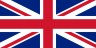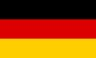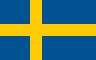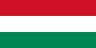Contents - Index

SQLite Functions

SQLite ships with its own inbuilt functions. They are grouped into 3 types. Core functions, Aggregate functions, and DateTime functions

In addition, the implementation of Sqlite that GSAK is using ships with the standard math functions of ACOS(), ASIN(), ATAN(), ATAN2(), CEIL(), CEILING(), COS(), COT(), DEGREES(), EXP(), FLOOR(), LN(), LOG(), LOG2(), LOG10(), MOD(), PI(), POW(), RADIANS(), SIGN(), SIN(), SQRT(), TAN(), TRUNCATE()

SQLite also provides the infrastructure to build custom functions that can be used in native SQL queries. GSAK has harnessed this power to add functions that are specific to GSAK. To prevent confusion with native/shipped SQLite functions, all GSAK functions begin with "g_"

Current GSAK functions include:

g_AttributeName(nId) : String - Returns the full attribute name of the corresponding attribute ID

g_Bearing1(sLat1,sLon1,sLat2,sLon2) : String - Calculates the cardinal bearing (N, NE, E, SE, etc) between two points

g_Bearing2(sLat1,sLon1,sLat2,sLon2) : Number - Calculates the bearing in degrees between two points

g_CacheType(sType) : String - Where sType1 is the 1 character cachetype abbreviation (which is how GSAK stores the cache type in the database). The return value is the expanded cache type description. This function would normally only be used on the "CacheType" field in the "Caches/CachesAll" table.

g_Chr(nChr) : String - Same as the macro Chr() function

g_CodeSort(sData) : String - Used internally by GSAK to sort the code column when the "special sort" option is selected. However, you can use this function to simulate the same sorting sequence for the waypoint code if required.

g_Contains(sFind,sData) : Boolean - MS Ansi Case insensitive search. For more information see SQLite Collation

g_DateFormat(sDate) : string  This function is virtually the same as the macro function DateFormat() but for use in Sql. This will enable Sqlite queries to display date fields formatted to the User's locale settings.

g_Distance(sLat1,sLon1,sLat2,sLon2,sUnits) : Real - calculate the distance between two points. sUnits can be K or M (K=Kilometers, M=Miles - see also the system variable \$_Units)  Assuming we have created a "caches" table with latitude and longitude fields and the records are sorted in the correct order, we can calculate the total distance in miles between all these waypoints with the following code:

 \$TotalDistance = SqLite("sql","SELECT sum(g_Distance(t1.latitude,t1.longitude,t2.latitude,t2.longitude,'M')) FROM caches AS t1, caches AS t2 WHERE t1.rowid = t2.rowid - 1")

Note: By default the calculation uses the more common Haversine forumlae (otherwise known as "Greater circle). However, the more accurate (but time consuming) high accuracy Vincenty formula is supported by appending a "H" to the units parameter. So if we wanted to use the Vincenty formulate in th example above the code would be:

 \$TotalDistance = SqLite("sql","SELECT sum(g_Distance(t1.latitude,t1.longitude,t2.latitude,t2.longitude,'MH')) FROM caches AS t1, caches AS t2 WHERE t1.rowid = t2.rowid - 1")

g_Elevation(rEle,[iRound]) : Real - This functions have been added to simplify usage of elevation. The elevation data stored in the database is *always* in meters. However, this function will take into account the user's settings and return the value as per the users miles/kms setting doing the conversion as required.This saves you having to worry about testing the \$_Units system variable and doing the corresponding conversion.

rEle - Elevation from database
iRound - Number of digits to round (optional, defaults to 1)

g_Equal(sData1,sData2) : Boolean - Test two strings for equality, ignoring case.

g_FormatCoordinates(sLatitude,sLongitude,sAction)  : string - enables a set of coordinates (in decima degree format, which is how GSAK databases store all coordinates) to be formatted in various different formats. sAction can be:

FormatBG - Format the input coordinates in British Grid.
FormatDegrees - Format the input coordinates in decimal degrees.
FormatMinutes - Format the input coordinates in decimal Minutes.
FormatSeconds - Format the input coordinates in decimal seconds.
FormatUTM - Format the input coordinates in UTM. sData should contain only one pair of coordinates.

g_FormatNumber(sFormatString,nNumber) : string  Format a number. For more information see the macro function FormatNumber()

g_FoundLog(sLogType) : Boolean - Use only with the "logs" table. The sole purpose is to return a Boolean to indicate the current log type is a "found" log or not.For example, instead of having to "hard wire" in the required log types to count all the find logs for a user as in:

 select count(*) from logs where lisowner and (ltype = 'Found it' or ltype='Attended' or ltype='Webcam Photo Taken')

We can now simply code as:

 select count(*) from logs where lisowner and g_foundlog(ltype)

Using this GSAK function in Sqlite macro code has several advantages:

a) Avoids typos and hard wiring the required log types
b) We don't have to remember what those log types are any more
c) Future proof macros, so will automatically work if Groundspeak decided to add a new "Found log" type. This of course would required a GSAK update, but at least we won't have to go back and change all macros that use this function.

g_GcDate(sLogDate,sLogTime) : string (YYYY-MM-DD)

The date that the Groundspeak site shows for logs is based on PST (Pacific Standard Time). However, the Date that is provided in the GPX file is GMT. This function will interrogate the given log date and time and then calculate the corresponding Groundspeak Date.

Unfortunately due to the "inconsistencies" of the Groundspeak date/times provided in the GPX file it is difficult to guarantee this date will precisely match Groundspeak. However, after a fair bit analyzing different logs in different years I have included a "kludge" in the algorithm that appears to work for all dates.

I would suggest that all macro authors use this function when they need a log date that corresponds to the same date that shows on the Groundspeak site.

An example of macro code to list all dates that differ:

 \$data = sqlite("sql","select lparent,ltype ,lby ,ldate,ltime,g_gcdate(ldate,ltime) as GcDate from logs where ldate <> gcdate","Headings=Y") \$data = sqltohtml(\$data,"Log dates different to Groundspeak","Y"

This will open your browser with a report similar to: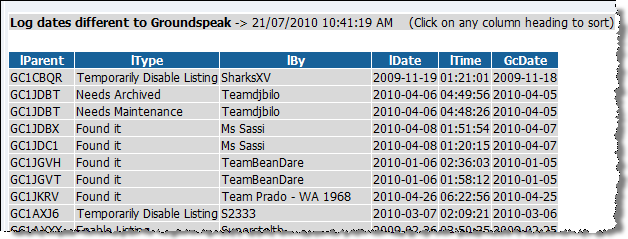g_GsCodeToNum: String - Convert a Geocaching.com code (GCGCH0C5 or TB4AZ9P etc) to the correspoinding ID number. This is the same as the native macro function GsCodeToNum()

g_IsValidDate(sDate) : Boolean - Tests if sDate is a valid SQLite date in the format YYYY-MM-DD

g_Left(sData,nLength) : String - Same as the macro left() function

g_LatLonFormat(sLatLon,sId) : String - Format the latitude or longitude in Minutes or seconds format. sLatLon must be one of Latitude or Longitude in decimal degree format (which is how the GSAK database stores lat and lon). sId identifies the transformation - latm = latitude minutes, lats = latitude seconds, lonm = longitude minutes, lons = longitude seconds. for example:

 \$out = sqlite("sql","select code,name, g_LatLonFormat(latitude,'latm') as Latitude,  g_LatLonFormat(Longitude,'lonm') as Longitude, Distance from caches ","Headings=Y") \$result = sqltohtml(\$out,"Coordinates formatted in minutes","Y")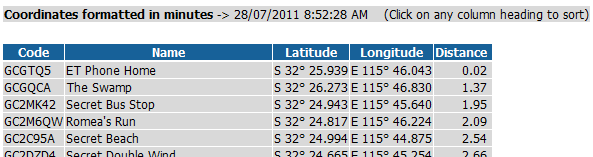g_NameSort(sData) : String - Used internally by GSAK to sort the waypoint name when the option "Special Sort" is selected. However, you can use this function to simulate the same sorting sequence for the waypoint name if required.

g_NumToGsCode: String - Convert a geocaching.com ID number to the corresponding code. This is the same as the native macro function NumToGsCode()

g_Project(sLatitude,sLongitude,nDegrees,nDistance,sUnits,sCalcType) : String - Project a waypoint

sLatitude - Latitude, must always be decimal degree format
sLongitude - Longitude, must always be decimal degree format
nDegrees - Degrees for projection
nDistance - Distance for projection (as per sUnits)
sUnits - Distance type, K = Kilometers, N = Nautical miles, M = miles, T = Meters, Y = Yards, F = Feet
sCalcType - G = Greater circle, V = Vincenty (High accuracy)

The return result is the projected coordinate, which is in decimal degree format and the latitude is separated from the longitude by a ; (semicolon).

g_Regex(sRegex,sData) : Boolean - Matching using regular expressions.Same definition and syntax as used in the native macro function RegEx()

g_RegexCount(sRegex,sData) : Integer - Count the number of times a regular expression is matched. Same definition and syntax as used in the native macro function RegExCount()

g_RegExData(sRegex, sData, nNumber) : String - Use this function to return the matched data instance of sRegex found in sData. Same definition and syntax as used in the native macro function RegExData()

g_RegexReplace(sRegex,sData,sReplaceWith,sBackRefSymbol) : String - String replace using Regex. Same definition and syntax as used in the native macro function RegExReplace()

g_Right(sData,nLength) : String - Same as the macro Right() function

g_SortBearing(sBearing) : String - Returns an "intuitive" sort sequence for Cardinal bearings (N, NE, E, SE, S, etc). That is, the bearing will be sorted in the same order as per a compass.

g_SortLatorLon(sLatLon) : String - Returns an "intuitive" sort sequence for Latitude and longitude, instead of purely numerical.

g_Upper(sData) : String - Return the MS ANSI uppercase string of sData. For more information see SQLite Collation